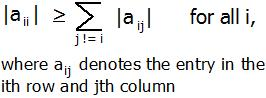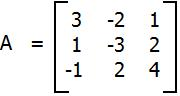Skip to content
Related Articles
Diagonally Dominant Matrix
• Difficulty Level : Easy
• Last Updated : 09 Apr, 2021

In mathematics, a square matrix is said to be diagonally dominant if for every row of the matrix, the magnitude of the diagonal entry in a row is larger than or equal to the sum of the magnitudes of all the other (non-diagonal) entries in that row. More precisely, the matrix A is diagonally dominant ifFor example, The matrixis diagonally dominant because
|a11| ≥ |a12| + |a13| since |+3| ≥ |-2| + |+1|
|a22| ≥ |a21| + |a23| since |-3| ≥ |+1| + |+2|
|a33| ≥ |a31| + |a32| since |+4| ≥ |-1| + |+2|
Given a matrix A of n rows and n columns. The task is tho check whether matrix A is diagonally dominant or not.
Examples :

```Input : A = { { 3, -2, 1 },
{ 1, -3, 2 },
{ -1, 2, 4 } };
Output : YES
Given matrix is diagonally dominant
because absolute value of every diagonal
element is more than sum of absolute values
of corresponding row.

Input : A = { { -2, 2, 1 },
{ 1, 3, 2 },
{ 1, -2, 0 } };
Output : NO```

The idea is to run a loop from i = 0 to n-1 for the number of rows and for each row, run a loop j = 0 to n-1 find the sum of non-diagonal element i.e i != j. And check if diagonal element is greater than or equal to sum. If for any row, it is false, then return false or print “No”. Else print “YES”.

## C++

 `// CPP Program to check whether given matrix``// is Diagonally Dominant Matrix.``#include ``#define N 3``using` `namespace` `std;` `// check the given given matrix is Diagonally``// Dominant Matrix or not.``bool` `isDDM(``int` `m[N][N], ``int` `n)``{``    ``// for each row``    ``for` `(``int` `i = 0; i < n; i++)``   ``{       ` `        ``// for each column, finding sum of each row.``        ``int` `sum = 0;``        ``for` `(``int` `j = 0; j < n; j++)            ``            ``sum += ``abs``(m[i][j]);       ` `        ``// removing the diagonal element.``        ``sum -= ``abs``(m[i][i]);` `        ``// checking if diagonal element is less``        ``// than sum of non-diagonal element.``        ``if` `(``abs``(m[i][i]) < sum)``            ``return` `false``;``       ` `    ``}` `    ``return` `true``;``}` `// Driven Program``int` `main()``{``    ``int` `n = 3;``    ``int` `m[N][N] = { { 3, -2, 1 },``                    ``{ 1, -3, 2 },``                    ``{ -1, 2, 4 } };` `    ``(isDDM(m, n)) ? (cout << ``"YES"``) : (cout << ``"NO"``);` `    ``return` `0;``}`

## Java

 `// JAVA Program to check whether given matrix``// is Diagonally Dominant Matrix.``import` `java.util.*;` `class` `GFG {``    ` `    ``// check the given given matrix is Diagonally``    ``// Dominant Matrix or not.``    ``static` `boolean` `isDDM(``int` `m[][], ``int` `n)``    ``{``        ``// for each row``        ``for` `(``int` `i = ``0``; i < n; i++)``        ``{       ``     ` `            ``// for each column, finding``            ``//sum of each row.``            ``int` `sum = ``0``;``            ``for` `(``int` `j = ``0``; j < n; j++)            ``                ``sum += Math.abs(m[i][j]);       ``     ` `            ``// removing the diagonal element.``            ``sum -= Math.abs(m[i][i]);``     ` `            ``// checking if diagonal element is less``            ``// than sum of non-diagonal element.``            ``if` `(Math.abs(m[i][i]) < sum)``                ``return` `false``;``       ` `        ``}` `        ``return` `true``;``    ``}` `    ``/* Driver program to test above function */``    ``public` `static` `void` `main(String[] args)``    ``{``        ``int` `n = ``3``;``        ``int` `m[][] = { { ``3``, -``2``, ``1` `},``                      ``{ ``1``, -``3``, ``2` `},``                      ``{ -``1``, ``2``, ``4` `} };``     ` `        ``if` `(isDDM(m, n))``             ``System.out.println(``"YES"``) ;``        ``else` `            ``System.out.println(``"NO"``);``    ` `    ``}``}` `// This code is contributed by  Arnav Kr. Mandal.`

## Python3

 `# Python Program to check``# whether given matrix is``# Diagonally Dominant Matrix.` `# check the given given``# matrix is Diagonally``# Dominant Matrix or not.``def` `isDDM(m, n) :` `    ``# for each row``    ``for` `i ``in` `range``(``0``, n) :        ``    ` `        ``# for each column, finding``        ``# sum of each row.``        ``sum` `=` `0``        ``for` `j ``in` `range``(``0``, n) :``            ``sum` `=` `sum` `+` `abs``(m[i][j])    ` `        ``# removing the``        ``# diagonal element.``        ``sum` `=` `sum` `-` `abs``(m[i][i])` `        ``# checking if diagonal``        ``# element is less than``        ``# sum of non-diagonal``        ``# element.``        ``if` `(``abs``(m[i][i]) < ``sum``) :``            ``return` `False` `    ``return` `True` `# Driver Code``n ``=` `3``m ``=` `[[ ``3``, ``-``2``, ``1` `],``    ``[ ``1``, ``-``3``, ``2` `],``    ``[ ``-``1``, ``2``, ``4` `]]` `if``((isDDM(m, n))) :``    ``print` `(``"YES"``)``else` `:``    ``print` `(``"NO"``)` `# This code is contributed by``# Manish Shaw(manishshaw1)`

## C#

 `// C# Program to check whether given matrix``// is Diagonally Dominant Matrix.``using` `System;` `class` `GFG {``    ` `    ``// check the given given matrix is Diagonally``    ``// Dominant Matrix or not.``    ``static` `bool` `isDDM(``int` `[,]m, ``int` `n)``    ``{``        ``// for each row``        ``for` `(``int` `i = 0; i < n; i++)``        ``{``    ` `            ``// for each column, finding``            ``//sum of each row.``            ``int` `sum = 0;``            ``for` `(``int` `j = 0; j < n; j++)        ``                ``sum += Math.Abs(m[i, j]);    ``    ` `            ``// removing the diagonal element.``            ``sum -= Math.Abs(m[i, i]);``    ` `            ``// checking if diagonal element is less``            ``// than sum of non-diagonal element.``            ``if` `(Math.Abs(m[i,i]) < sum)``                ``return` `false``;``        ` `        ``}` `        ``return` `true``;``    ``}` `    ``// Driver program``    ``public` `static` `void` `Main()``    ``{``        ``int` `n = 3;``        ``int` `[,]m = { { 3, -2, 1 },``                    ``{ 1, -3, 2 },``                    ``{ -1, 2, 4 } };``    ` `        ``if` `(isDDM(m, n))``            ``Console.WriteLine(``"YES"``) ;``        ``else``            ``Console.WriteLine(``"NO"``);``    ` `    ``}``}` `// This code is contributed by Vt_m.`

## PHP

 ``

## Javascript

 ``

Output :

`YES`

Attention reader! Don’t stop learning now. Get hold of all the important DSA concepts with the DSA Self Paced Course at a student-friendly price and become industry ready.  To complete your preparation from learning a language to DS Algo and many more,  please refer Complete Interview Preparation Course.

In case you wish to attend live classes with industry experts, please refer DSA Live Classes

My Personal Notes arrow_drop_up